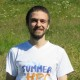Electrons in a nanotubeSOLID2000 is a simulation program for systems that have symmetry in three dimensions, such as crystals. The code is written mostly in FORTRAN77 and FORTRAN95.

My project is to parallelize the calculation of the band structure using MPI, which is basically the energy of the electrons inside the solid. Such solutions are impossible to find analytically for the real word systems, that’s why we have to use numerical methods to sample part of our solution.

The finest (dense) our sampling is, the better our plot will be, and the more calculation time is required. For every sample we make, we have to solve an NxN matrix, i.e to find its eigenvalues. Those eigenvalues are the solution we desire, i.e the energy of the electrons, and the matrix is called the Hamiltonian matrix which describes the whole energy of our system, potential energy + the kinetic energy. Normally the size of the matrix is infinite so it’s up to “cut” the matrix.

The time required to calculate the eigenvalues scales as N3  and therefore we want our matrix to be as small as possible but not too small because the accuracy of our results will be very low. Our strategy to parallelize the band structure is to assign every sampling to a procedure up until all the sample points are done.

This will be done by a message that the master will send to all the slaves to signal that the band structure calculation is about to start and each procedure will calculate its sample points. The work will be distributed equally throughout the procedures in order to have the maximum efficiency. At the end of the calculation, the slaves must send their samples to the master so the master can write the band structure in a file. The next picture shows an example of a band structure of a carbon nanotube which was generated using this code.Band structure of a carbon nanotube where y-axis is the Energy and x-axis is the k-point (or the sample point)Carbon nanotubeI finished my BSc in physics department of AUTH University of Greece. During my bachelor any kind of programming was my hobby. Doing my bachelor thesis in computational solid stated physics, gave me the opportunity to start working on programming more seriously. After my graduate I jumped to my master, "Computational Physics" in which I learned many different numerical methods applied to various fields of physics.

Tagged with: , , , , , , , ,

This site uses Akismet to reduce spam. Learn how your comment data is processed.